# Procedural World - Textures

I’m getting to the point of adding more detail into the world. Up to this point blocks only have a single color and of course this isn’t very flexible.

It’s time to add textures to the block meshes!

The way I have this setup is I have created a texture atlas that I imported into unity. The atlas contains 16x16 sprites representing faces for each block. I use unity’s sprite editor to splice up the atlas into indiviual sprites. Now I’m not using Unity sprites directly. I’m using the Sprite.uv field to grab the uv coordinates for individual faces.

Ok! Now it’s just a matter of mapping the textures on the faces!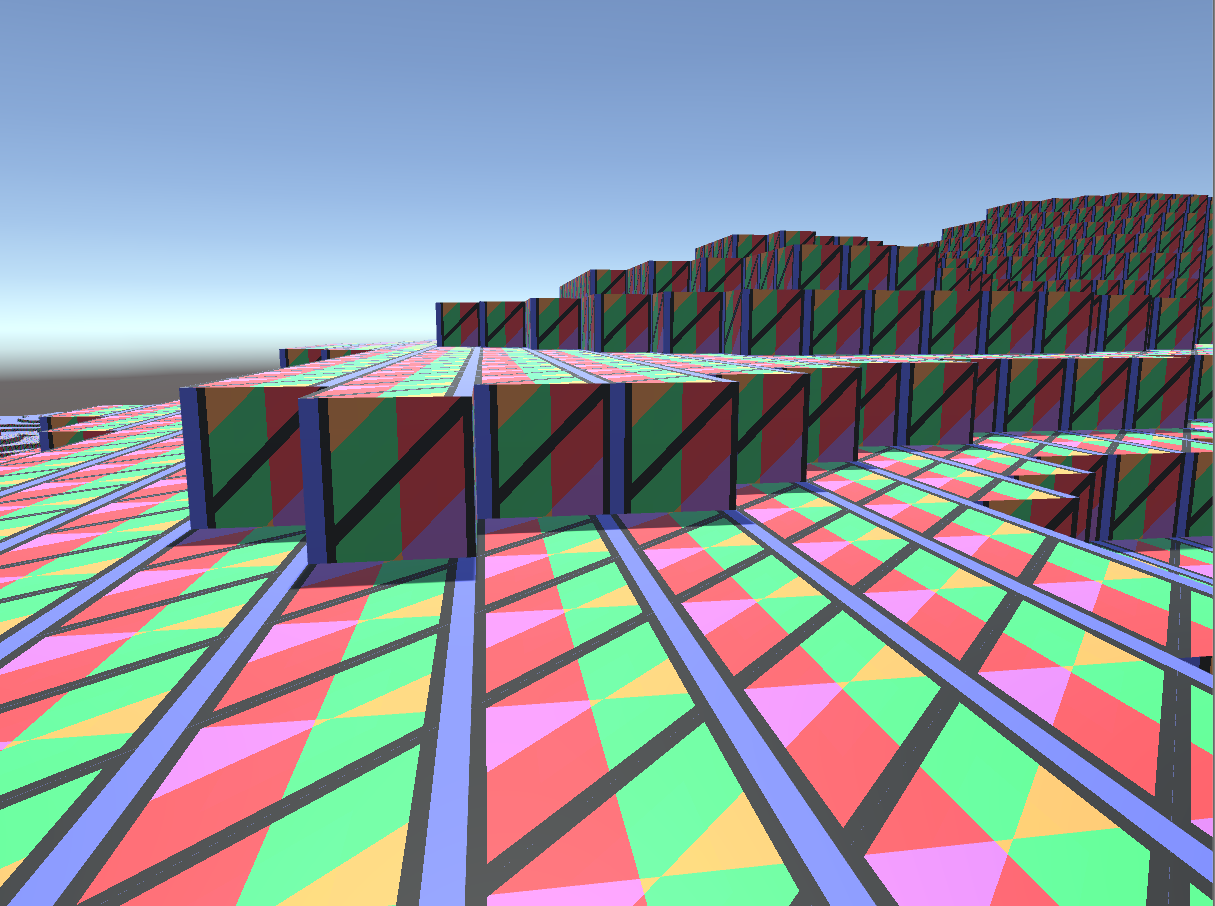Well that isn’t right. The problem is I have ordered the vertices in the mesh clockwise started at the bottom left corner. Unity’s sprite.uv is ordered differently.

Ok so reorder them.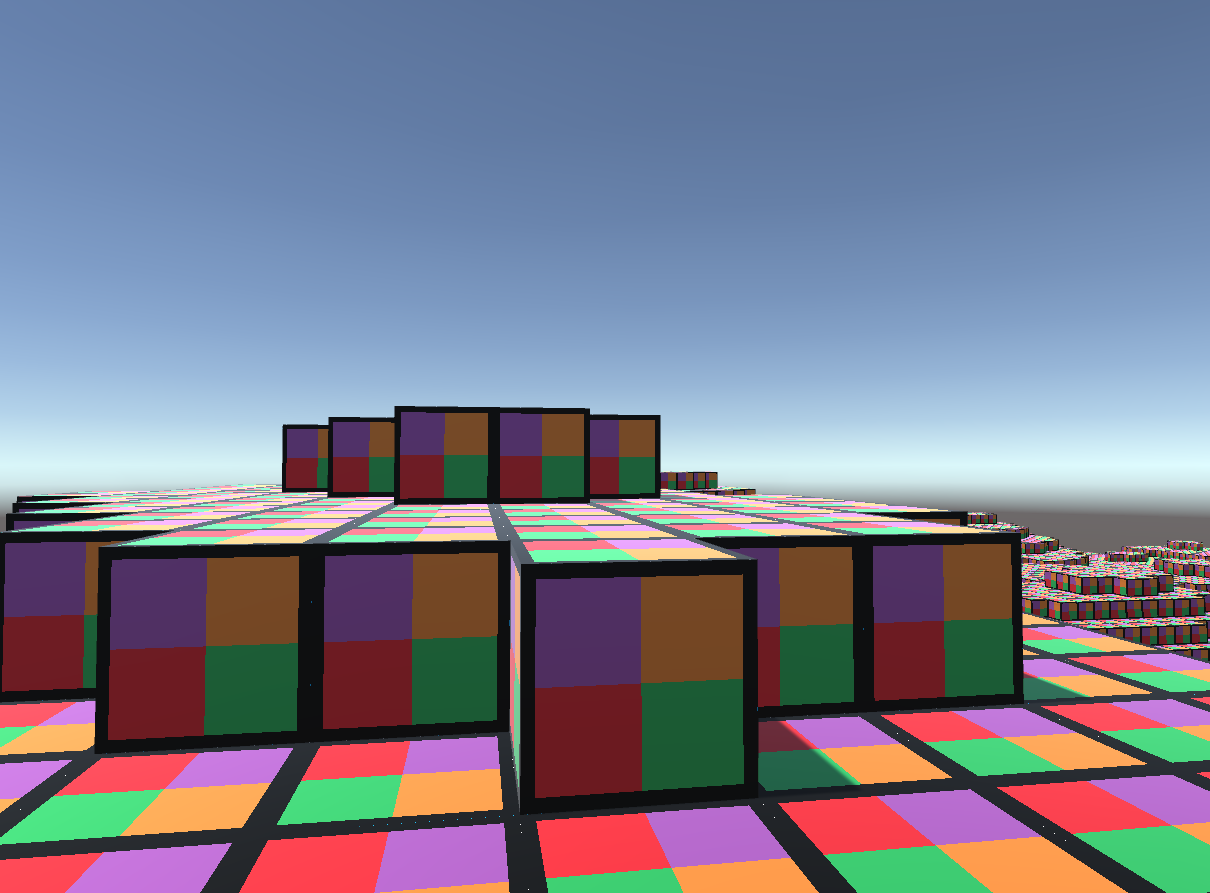Awesome. But the problem is this is using the naive mesher. Also the oriental of the face can to confusing with the current texture so I changed that up.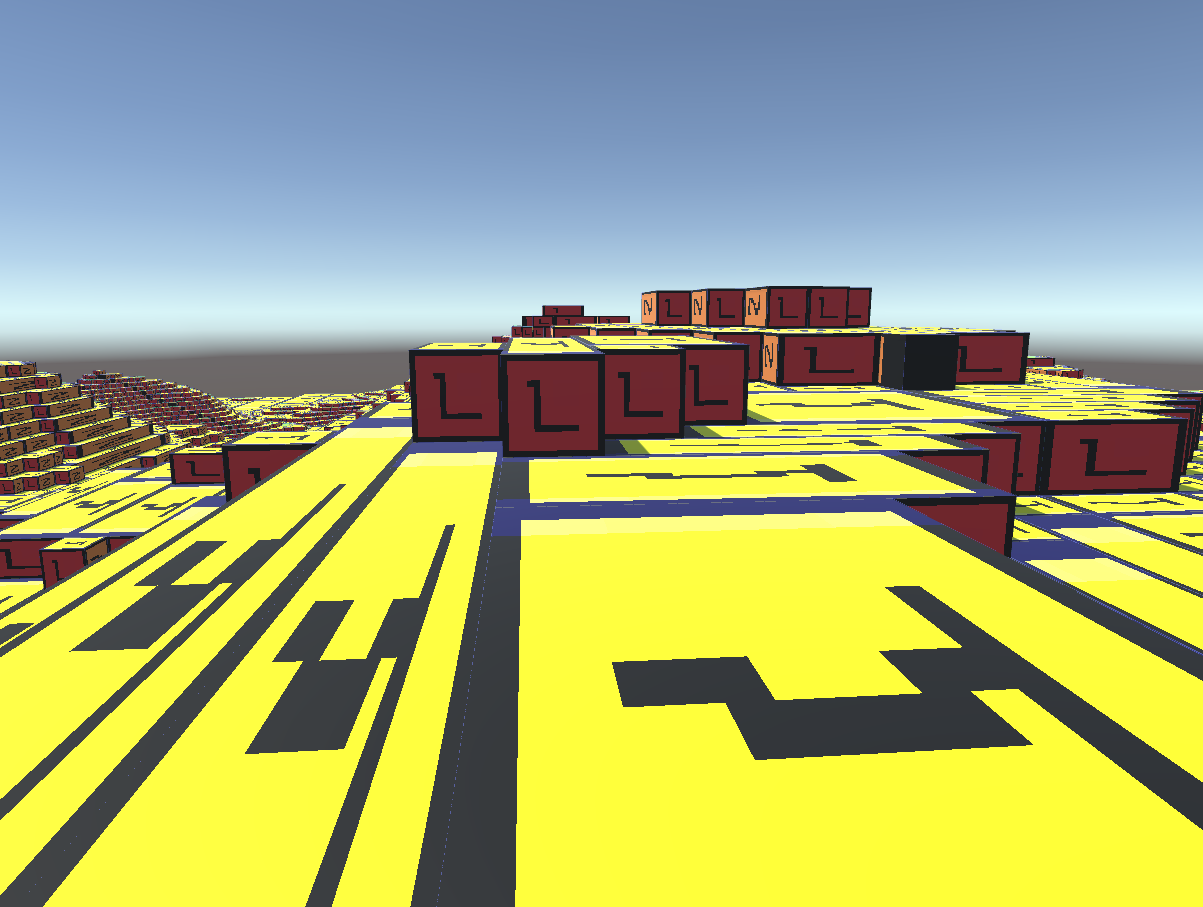This is what happens using the greedy mesher. The uvs get streched across the face quad. There wasn’t really an easy to fix this in the mesh generation logic.

So what we need to do is ensure the texture tiles as much as needed while rendering across a large quad.

Shader "Custom/Block" {
Properties {
_BlockAtlas ("Block Atlas Texutre", 2D) = "white" {}
_Glossiness ("Smoothness", Range(0,1)) = 0.5
_Metallic ("Metallic", Range(0,1)) = 0.0
_TileSize ("Tile Size", Range(0,1)) = 0.0
_Offset ("Alignment Adjustment", Float) = 0.0
}
Tags { "RenderType"="Opaque" }
LOD 200

CGPROGRAM
// Physically based Standard lighting model, and enable shadows on all light types

// Use shader model 3.0 target, to get nicer looking lighting
#pragma target 3.0

struct Input {
float3 worldPos;
float3 worldNormal;
float2 uv_BlockAtlas;
float4 color : COLOR;
};

sampler2D _BlockAtlas;

half _Glossiness;
half _Metallic;
half _TileSize;
half _Offset;

// get u direction (y axis) from normal
float3 getU(int n) {
if (n == 0 || n == 1) {
return float3(0, 1, 0);
}
else if (n == 2) {
return float3(0, 0, 1);
}
else if (n == 3) {
return float3(0, 0, -1);
}
else if (n == 4 || n == 5) {
return float3(0, 1, 0);
}
else {
return float3(0, 0, 0);
}
}

// get the V direction (x axis) from the normal
float3 getV(int n) {
if (n == 0) {
return float3(0, 0, -1);
}
else if (n == 1) {
return float3(0, 0, 1);
}
else if (n == 2 || n == 3) {
return float3(1, 0, 0);
}
else if (n == 4) {
return float3(1, 0, 0);
}
else if (n == 5) {
return float3(-1, 0, 0);
}
else {
return float3(0, 0, 0);
}
}

// get the face the normal represents
int getType(float3 n) {
if (n.x != 0) {
if (n.x < 0) {
return 0;
}
else {
return 1;
}
}
else if (n.y != 0) {
if (n.y > 0) {
return 2;
}
else {
return 3;
}
}
else if (n.z != 0) {
if (n.z < 0) {
return 4;
}
else {
return 5;
}
}
else {
return 6;
}
}

void surf (Input IN, inout SurfaceOutputStandard o) {

float3 offset = float3(0, 0, 1) * _Offset;
float3 position = IN.worldPos + offset;

float3 normal = IN.worldNormal;

// bottom left corner of the tile is passed to the mesh as a uv
float2 tileOffset = IN.uv_BlockAtlas;
float2 tileSize = float2(_TileSize, _TileSize);

int nt = getType(normal);
float3 du = getU(nt);
float3 dv = getV(nt);

float2 tileUV = float2(dot(dv, position), dot(du, position));
float2 texCoord = tileOffset + (tileSize * frac((tileUV)));

// sample the texture atlas at the calculated position.
fixed4 c = tex2D (_BlockAtlas, texCoord);

o.Albedo = c.rgb;
o.Alpha = c.a;

// Metallic and smoothness come from slider variables
o.Metallic = _Metallic;
o.Smoothness = _Glossiness;
}

ENDCG
}
FallBack "Diffuse"
}



This shader gets the 2 perpendicular axes or the face from the normal vector. Then calculates the texture coordinate given the size of the tile, the uv offset provided to the mesh and fractional component of the world space position of the fragment.

Correct orientation and tiling.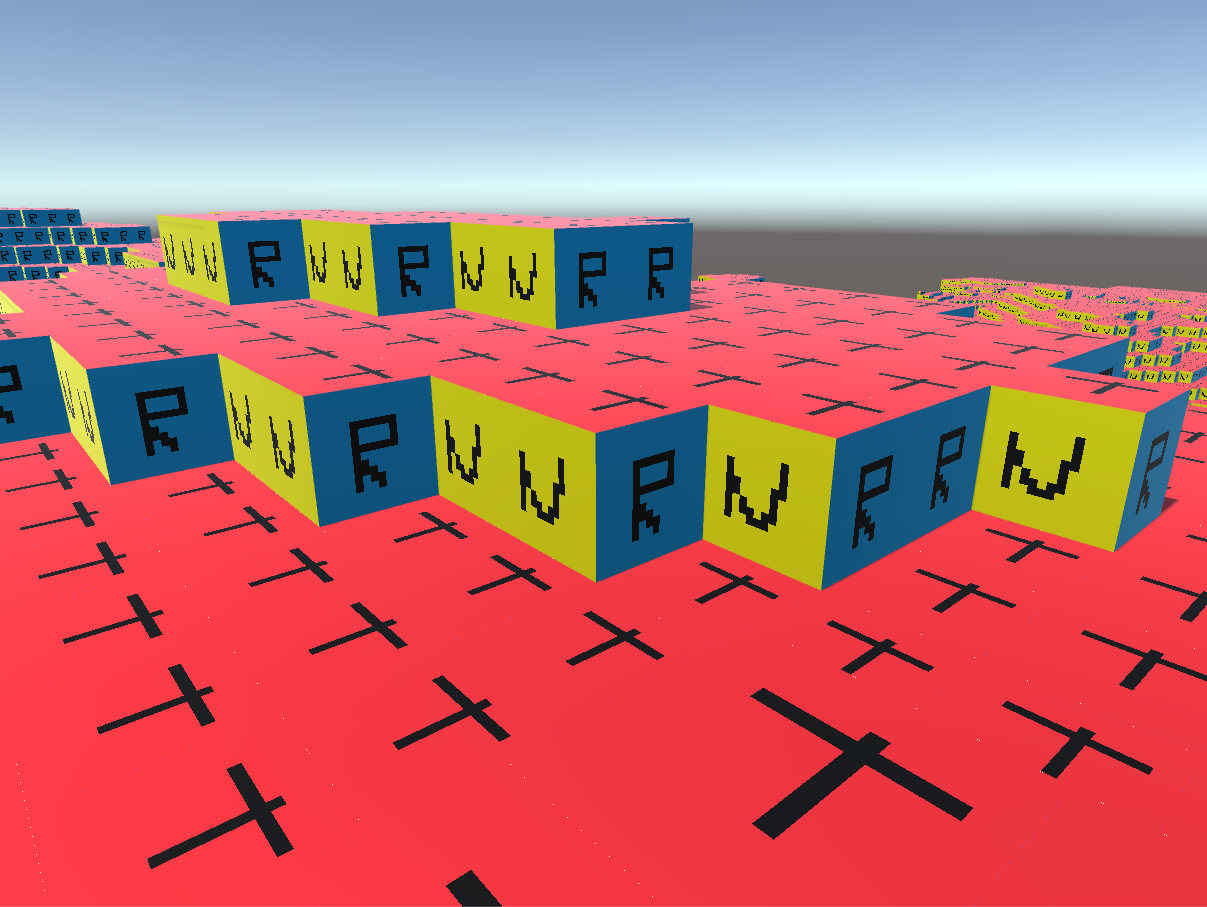With Minecraft textures: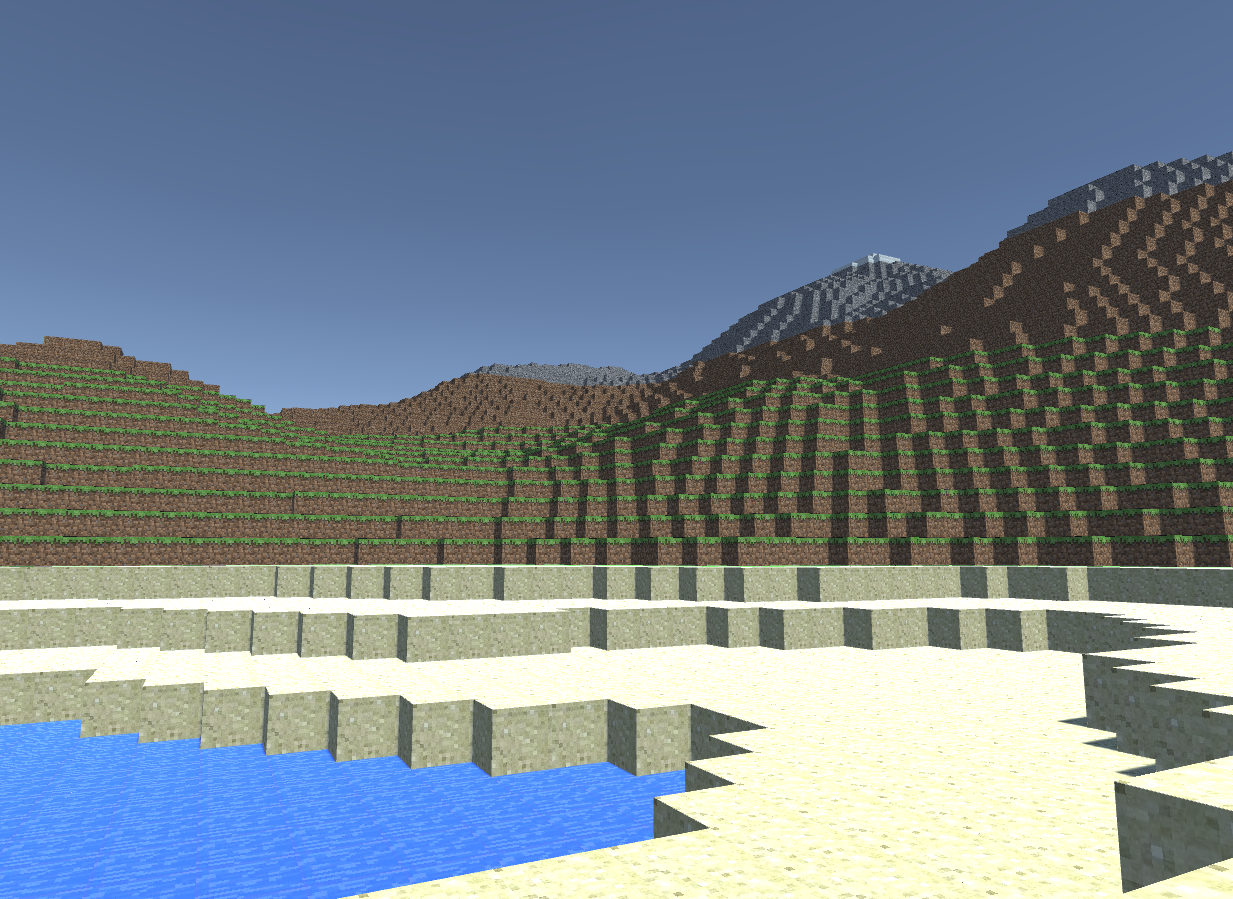Now I feel the shader is kind of brute forcey. So I will try to improve it at a later time.